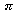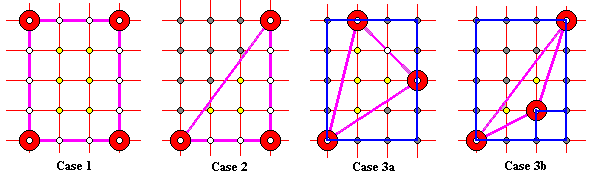# Pick's Theorem### Theorem

Let P be a lattice polygon. Assume there are I(P) lattice points in the interior of P, and B(P) lattice points on its boundary. Let A(P) denote the area of A. Then

A(P) = I(P) + B(P)/2 - 1

### Proof

For every lattice point p in the interior or boundary of P, let ap denote the angle of visibility of P from p. For points in the interior of P, ap = 2. For points on the boundary other than vertices, ap = π. For a vertex p, ap is just the internal angle of the polygon at that vertex. Let wp = ap/2π, and introduce W(P) =wp, where the sum is taken over all lattice points either inside or on the boundary of P. Then

 (1) W(P) = A(P)

The proof of (1) proceeds in three steps. Firstly, note that function W is additive. Combining two polygons that share a piece of boundary into one either replaces two boundary points with one interior point or, when the point remains a vertex, adds up the two internal angles at that vertex, one from each polygon.Secondly, verify (1) for simple shapes: lattice rectangle (Case 1) with sides parallel to the grid lines, half lattice rectangle (Case 2), and arbitrary lattice triangle where we have to consider a couple of cases (Cases 3a-b.) Embed the triangle into the smallest possible rectangle from Case 1. Such a rectangle will naturally appear as a union of the given triangle and pieces from the two previous cases. Apply additivity.

Thirdly, show that every simple lattice polygon may be dissected into a union of lattice triangle (e.g., by its diagonals.)

Acquipped with (1), we have only to compute W(P). Let P has n vertices and define b = B(P) - n. The sum of internal angles of P is (n - 2)*. At all other boundary points p (whose number is b), ap = π. Therefore,wp, where the sum is taken only over boundary points is (n - 2)/2 + b/2 = (n + b)/2 - 1 = B(P)/2 - 1. Adding the sumwp over the internal points which is simply I(P) completes the expression for W(A):

W(P) = I(P) + B(P)/2 - 1

This combined with (1) proves the theorem.